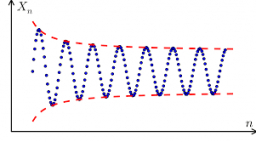# Geometric 61524

Determine the first term and quotient in the geometric sequence: a4 = -8 / 3; a6 = -32 / 3

q =  2
a1 =  -1/3

### Step-by-step explanation:Did you find an error or inaccuracy? Feel free to write us. Thank you!

Tips for related online calculators
Need help calculating sum, simplifying, or multiplying fractions? Try our fraction calculator.